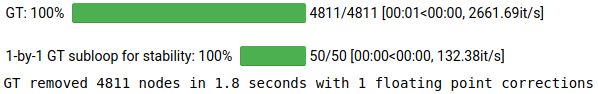# PyGT - Graph transformation and reduction in Python¶

Graph transformation is designed for the analysis of highly metastable (ill-conditioned) Markov chains, where linear algebra methods fail. [Wales09]

PyGT produces stable coarse-grained models with exact branching probabilities and mean first passage times, in discrete or continuous time. [Swinburne20a]

Note

You can install PyGT using the pip package manager (preferably in a virtual environment):

pip install PyGT

Simplest possible usage in continuous time with transition rates $$k_{ij}$$:
• Vector tau of mean state waiting times $$\tau_j=1/\left(\sum_ik_{ij}\right)$$
• Sparse or dense matrix B of branching probabilities $$B_{ij}=k_{ij}\tau_j$$
• Boolean vector rm_vec selecting nodes to remove
import PyGT
# Removes nodes in blocks of <=50 whilst retaining numerical stability
gt_B, gt_tau = PyGT.GT.blockGT(rm_vec,B,tau,block=50,screen=True)Note

Tutorials (see menu) can be run online with binder:The notebooks can also be cloned from the PyGT github repo:

# clone entire source code and examples
git clone https://github.com/tomswinburne/PyGT.git
# go to examples folder
cd PyGT/examples
# run notebook
jupyter-notebook basic-functions.ipynb


Graph transformation [Wales09] is a deterministic dimensionality reduction algorithm that iteratively removes nodes from a Markov Chain while preserving the mean first passage time (MFPT) and branching probabilites between the retained nodes. The original Markov chain does not need to satisfy detailed balance.

This package provides an efficient implementation of the graph transformation algorithm, accelerated via partial block matrix inversions [Swinburne20a] for abitrary discrete-time or continuous-time Markov chains [Kannan20a]. Code is also provided for the calculation of first passage time statistics and phenomenological rate constants between endpoint macrostates [Wales09] [Swinburne20b].

We also include code for two different approaches to the dimensionality reduction of Markov chains using the graph transformation algorithm. In the first approach, we consider the problem of estimating a reduced Markov chain given a partitioning of the original Markov chain into communities of microstates (nodes) [Kannan20a]. Various implementations of the inter-community rates are provided, including the simplest expression given by the local equilibrium approximation, as well as the optimal rates originally derived by Hummer and Szabo. In the second approach, which we call partial graph transformation [Swinburne20a] [Kannan20b], select nodes that contribute the least to global dynamics are renormalized away with graph transformation. The result is a smaller-dimensional Markov chain that is better-conditioned for further numerical analysis.

All methods are discussed in detail in the following manuscripts, which should also be cited when using this software:

References

 [Wales09] (1, 2, 3) D.J. Wales, Calculating rate constants and committor probabilities for transition networks by graph transformation, J. Chemical Physics (2009), https://doi.org/10.1063/1.3133782
 [Swinburne20a] (1, 2, 3) T.D. Swinburne and D.J. Wales, Defining, Calculating, and Converging Observables of a Kinetic Transition Network, J. Chemical Theory and Computation (2020), https://doi.org/10.1021/acs.jctc.9b01211
 [Swinburne20b] T.D. Swinburne, D. Kannan, D.J. Sharpe and D.J. Wales, Rare Events and First Passage Time Statistics From the Energy Landscape, Submitted to J. Chemical Physics (2020)
 [Kannan20a] (1, 2) Kannan, D.J. Sharpe, T.D. Swinburne and D.J. Wales, Dimensionality reduction of Markov chains using mean first passage times with graph transformation, In Prep. (2020)
 [Kannan20b] Kannan, D.J. Sharpe, T.D. Swinburne and D.J. Wales, Dimensionality reduction of complex networks with graph transformation, In Prep. (2020)